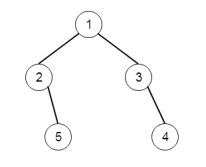# Program to perform level order traversal of binary tree in C++

Suppose we have a binary tree. We have to traverse this tree using level order traversal fashion. So if the tree is likeThe traversal sequence will be like: [1,2,3,5,4]

To solve this, we will follow these steps −

• define queue que to store nodes

• insert root into the que.

• while que is not empty, do

• item := item present at front position of queue

• print the value of item

• if left of the item is not null, then insert left of item into que

• if right of the item is not null, then insert right of item into que

• delete front element from que

Let us see the following implementation to get better understanding −

## Example

Live Demo

#include <bits/stdc++.h>
using namespace std;
void print_vector(vector<int> v){
cout << "[";
for(int i = 0; i<v.size(); i++){
cout << v[i] << ", ";
}
cout << "]"<<endl;
}
class TreeNode{
public:
int val;
TreeNode *left, *right;
TreeNode(int data){
val = data;
left = right = NULL;
}
};
class Solution {
public:
vector<int> solve(TreeNode* root) {
if(!root)
return {};
vector <int> ret;
queue <TreeNode*> q;
q.push(root);
while(!q.empty()){
TreeNode* node = q.front();
q.pop();
ret.push_back(node->val);
if(node->left){
q.push(node->left);
}
if(node->right){
q.push(node->right);
}
}
return ret;
}
};
main(){
TreeNode *root = new TreeNode(1);
root->left = new TreeNode(2);
root->right = new TreeNode(3);
root->left->right = new TreeNode(5);
root->right->right = new TreeNode(4);
Solution ob;
print_vector(ob.solve(root));
}



## Input

TreeNode *root = new TreeNode(1);
root->left = new TreeNode(2);
root->right = new TreeNode(3);
root->left->right = new TreeNode(5);
root->right->right = new TreeNode(4);

## Output

[1, 2, 3, 5, 4, ]